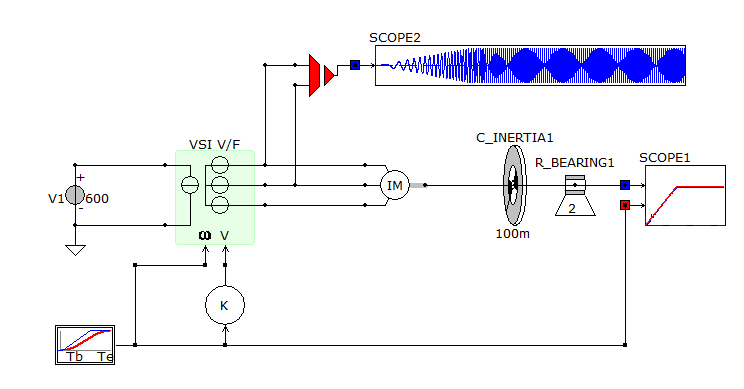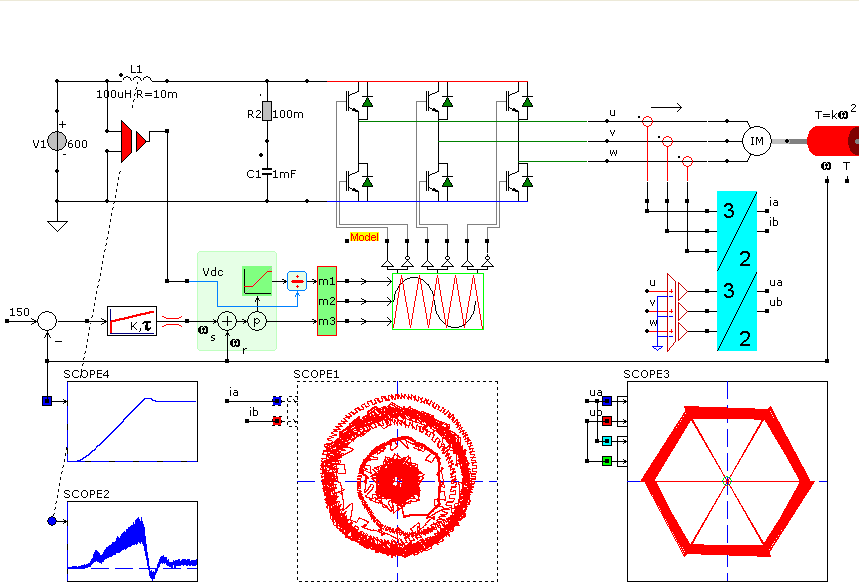﻿Caspoc Simulation & Animation for Power Electronics & Electric Drives

Study of V/f control operation of 3Phase Induction Motor drive

The Volt-Hertz control is he most simple yet very effectife way of controlling the speed of an induction machine. Ther reason for applying this circuit is the constant field in the motor.

In order to study the concept of the V/F control, as simple inverter component model including the V/F control is used. Here the VSI_V/F block models the votlage stiff inverter where the frequency and amplitude of the output votlage can be controlled. The connection on the left side should be connected to the DC link and at hte putput connection on hte rigth side an induction machine is connected. A simple mechanical load of J=100mKgm2 and friction of 2 [Nm/Rad/s] is used.

The ramping of hte frequency and voltage is done suning a signal block that provides the signal for the angular speed of the output votlages. The signal for controlling the amplitude votlages are made by multiplying the angular speed signal with gain K. If the start-up tmie is choosen long enough, the rotor speed will follow the angular speed signal.A more detailed implementation with PWM and complete inverter modeled is given below. Here the V/F control takes as inputs the DC link voltage Vdc(left-top), the measured angular rotor speed wr(bottom) and the proposed angular slip frequency ws(left-bottom). The angular slip frequency is determined based on difference between the reference and the measured angular rotor speed. This difference is inoput to a PI controller, whose output isthe angulrar slip frequency.

The output from the V/F control block are the dutycycles for the modulator. The ouput from the modulator are the gating signals for hte inverter. The DC link iinput current is measered in a paracitic inductance in the DC link. This series connection of inductance of 100uH anad 10 mOhm models the losses in the busbar.

The simualtion results are displayed where the measured currents and voltage to the induction machine are shown as space vectors. The current draws a circle with the radius equal to the amplitude and the voltage follwos a hexagon, becuase of the 6 possible igbt state combinations inside the inverter.A concetual model can be used to study the effects of the V/F control, wher it can be determined how fast the drive will start and what current level are reached. A more detailed model also reveals the hamronic content in the current